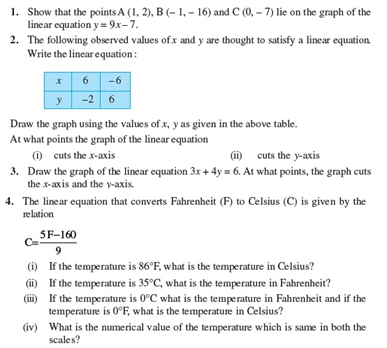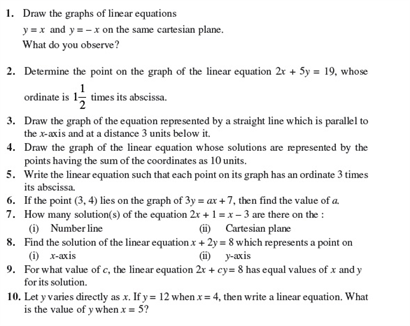# Revision Notes For CBSE Class 9 Math Chapter -4 Linear Equations in Two Variables

A Linear equation is an equation between the two variables which forms a straight line when plotted on a graph. The variables are the real numbers and the coefficients may be considered as parameters of the equation

The equation with a homogeneous variable is known as the Linear equation in one variable.

For e.g.: 4x = 9, then x = 9/4. This is an example of a Linear equation in one variable.

The equation with more than one variable is known as a Linear equation in two variables.

For e.g.: 5x+3y = 7. This is an example of the Linear equation in two variable.

A linear equation in two variable is the first-degree equation and it can be written in the form ax + by = c. Here, a and b should not be zero, and a, b, and c should be real numbers. The graph of any equation of this form is a straight line.

Check here for the free CBSE Class 9 Math revision notes for Chapter 4- Linear Equations in Two Variables.